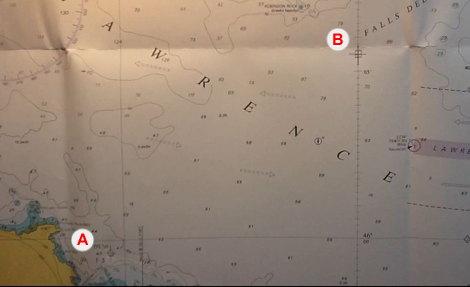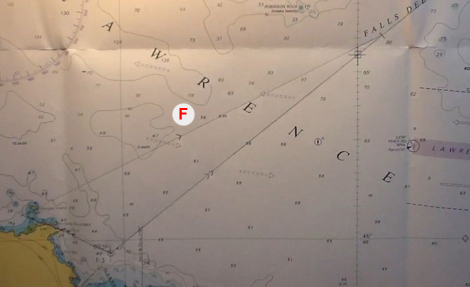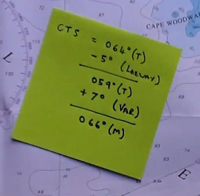# Course to steer (CTS) – DS & YM

## Preparation

Before reading this page you will need to have a copy of the training almanac and chart (and a real one if possible).

You will need to be happy calculating tidal streams, converting between magnetic and true north and to understand the principals of leeway.

## Why is this important?

If you head straight to your destination you may not arrive quite where you expected. This might be because the tide pushed you off course and/or the force of the wind pushing us downwind (leeway).

It is far easier, quicker and safer to allow for the tide and wind by pointing your boat in a direction that takes you directly to your destination.

One way to think about this is to imagine a fast flowing river (as in the video below), if you aim straight across you would will end up a long way down the river. To counter the river flow you need to aim across but also up the river.

In the video, you can see it is easy for the canoeist to visually judge the angle to point the canoe. At sea and over longer distances it is important to calculate in advance the direction to travel in order to counter the tide and wind.

### Why bother, doesn’t the GPS tell us which way to go?

It might be tempting to think the GPS will tell you which way to go, however GPS units are not programmed with tidal flows. So the best it can do is keep you on track based on the tide the boat is experiencing at that moment in time – it cannot predict the future. If you follow the GPS, assuming you are travelling fast enough to counter any tidal current and leeway, you will eventually reach your destination. There is no guarantee that the route you travel will be the quickest or indeed safe, this is why we should still calculate a CTS.

### Calculating a CTS

The easiest way to see how a Course to Steer (CTS) works is to work through an example.

On your sailing yacht the GPS gives a position of 45o 59.6’N 006o 10.5’W at 09:30, Log reading 1.3 (Point A on the picture). You wish to travel to a waypoint at 46o 05.5’N 006o 00.0’W (B).

You travel at a speed of 12 Knots with a tide running on a course of 293o at 2.9 Knots.First we draw a line from the current position through (and beyond) the position of the waypoint.We note that as the approximate distance of travel is 10Nm and we are travelling at 12Kt it will probably take just under 1 hour to complete this journey.

Therefore we will be effected by one hour of tide. To allow for this we draw a line representing one hour of tide, this line is drawn from the start position (C).As we will be travelling at 12kt for one hour will should travel a distance of 12Nm.

Therefore we set our dividers to 12Nm, placing one end of the dividers on the end of the tide line (D) and finding the point where the other end meets original line (E) – we mark this point.Draw a line from the end of the tidal line to the point we marked using the dividers.

Common Mistake: The most common mistake when calculating a CTS is to join the end of the tidal vector with the destination (ie. the waypoint in this case), instead of the point you have marked of on the line.Measure the bearing of this new line (F) using your plotter which gives us 064o(T).To see the whole method explained watch this video.

### Calculating the tidal stream

In a real life situation, you will not be simply given the tide speed and direction but will need to calculate it using the method shown on the tidal stream webpage.

Allowing for leeway, variation and deviationIf you are unsure what is meant by Leeway, see the Leeway page for more explanation.

The effect of leeway will always push you vessel ddownwind to allow for this we head however many degrees further upwind to counter this effect. it is therefore useful to draw a wind arrow on the chart and image the effect of the wind. You can then establish whether the leeway should be added or subtracted from your heading.

For CTS the rule is:
– Wind coming from Starboard – Add (Plus)
– Wind coming from Port – Subtract

Note: this is the opposite to DR

Once you have applied Leeway you need to apply Variation and then finally Deviation (Yachtmaster only). ie.

Water Track ± Leeway ± Variation ± Deviation = Course to Steer (C)

In the example above we might estimate leeway of 5o with the north westerly wind.

To counter this leeway we must steer 5o into the wind in other words 5ofurther north in this case. The CTS is therefore 064o – 5o = 059o(T).

We finally add 7o for variation as above give us a Course to Steer (CTS) of 066o(M). If you are not sure how to do this see the notes on magnetic north.

To see this explained watch this video.

### How long will it take

We may wish to know how long it will take to get from our start position to the location we wish to get to.

The precise method of doing this is to measure the distance we would have travelled in one hour (from A to B is 10.4Nm in this case) and the distance we actually want to travel (from A to C is 9.4Nm in this case).You then take the distance you wish to travel and divide be the one hour distance. 9.4 / 10.4 = 0.904.

You then multiply by 60 (as there are 60 minutes in an hour) which gives you 54 minutes (to the nearest minute). So it will take approximately 54 minutes to get from A to C.

To see the whole method explained watch this video.

Note: if you were doing this calculation for more than one hour (as below) to would multiply by the number of minutes in corresponding number of hours (ie. for two hours you would multiply by 120).

### Travelling for less than an hour

Sometimes it is clear that we will take far less than an hour to reach our destination. In this case it is possible to construct a CTS using half of one hours tide and distance.

To see this explained watch this video.

### Travelling for more than an hour

Sometimes it will be clear that it will take more than one hours tide to make it to your destination. In this case you can calculate a CTS using two or more hours or tide.

You use the same method with the following differences:
– The total tidal stream for the hours of travel would be plotted end to end.
– You arc would be for the total distance ie. if you travel at 6 Knots for 2 hours your would draw an arc of 12 Nm.

To see the whole method explained watch this video.

#### Video: skill (YM only)

Use this spreadsheet to check your calculations. You can also use this to create and check questions of your own. Remember you will not be able to use this in the test!

Course to Steer Examples
This is a link to a number of Course to Steer examples, with answers.

### YBW Guide